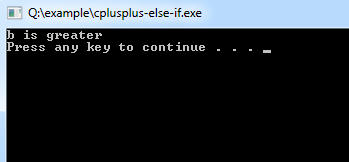C++ else-if Statements

else if statements in C++ is like another if condition, it's used in a program when if statement having multiple decisions.

The basic format of else if statement is:

Syntax:
if(test_expression)
{
}
else if(test_expression n)
{
}
else
{
}

Example of a C++ Program to Demonstrate else if Statement

Example:
#include <iostream>
using namespace std;

int main()
{
int a=15,b=20;

if(b>a)
{
cout << "b is greater"<<endl;
}else if(a>b){
cout << "a is greater"<<endl;
}
else
{
cout << "\n Both are equal"<<endl;
}
system("PAUSE");
}
Program Output: